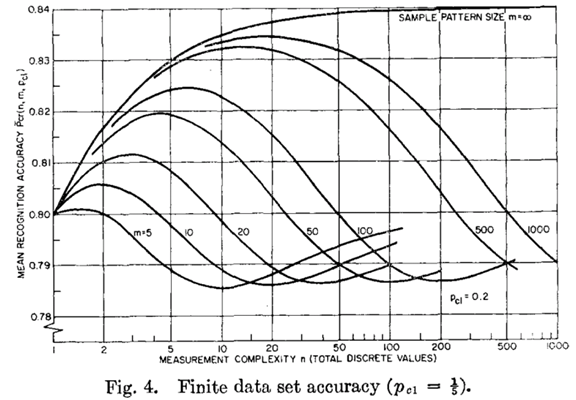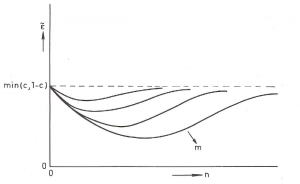The peaking paradox was heavily discussed in pattern recognition after a general mathematical analysis of the phenomenon was published by Hughes in 1968. It has puzzled researchers for at least a decade. This peaking is a real world phenomenon and it has been observed many times. Although the explanation by Hughes seemed general and convincing, it also raised questions.

In 1978 Van Campenhout beautifully explained the basic defects, but phrased the title of his paper as “The Resolution of an Apparent Paradox”. This was again confusing, as by showing that the basic model used by Hughes was inappropriate, also the explanation of the peaking paradox became invalid. As a result, the paradox itself still remained as such.

Until today many researchers refer to peaking as Hughes’ phenomenon or Hughes’ paradox in spite of the fact that the paper was wrong. Here, we will sketch the basic idea of the paper and some of the flaws it contains as described by Van Campenhout and by others.

### The expected recognition accuracy

Hughes observed that measurements have a finite accuracy. The outcome of any set of measurements may thereby be represented as a vector (point) in a finite, discrete space. As different measurements may lead to the same representation point, this point may also be understood as a cell in which all indistinguishable outcomes are collected. More features or a higher measurement accuracy will result in an outcome space with more cells. In a given problem these cells have a particular probability for the classes to be distinguished. A training set may be used to estimate these probabilities. On top of this, also the probability can be computed that a cell is assigned to the wrong class. This results in an expected classification error for a given problem, i.e. integrated over all possible training sets.

### The mean recognition accuracy

If we are interested in the performance of classification error as a function of increasing problem complexity (e.g. more measurement cells)  then sets of problems have to be considered.  This involves a probability distribution over problems. Hughes modeled this by a uniform prior: for any measurement space all class probabilities for all cells are equally likely, provided, of course, that the sum of probabilities is one when appropriate.

The result of the analysis, called the mean recognition accuracy (‘mean’ refers to the average over the set of problems) is given by the above  figure. It holds for two-class problems with the (0.2, 0.8) class priors. In the horizontal direction the number of cells in the space is plotted and in the vertical direction the resulting recognition accuracy. Different curves are shown for different sizes of the training set. These curves show a maximum: for more complex measurement spaces the recognition accuracy deteriorates after some optimal complexity.

### What is wrongThe main peaking phenomenon as observed in practical situations is visible in Hughes’ figures. There is, however, a strange overshoot phenomenon. The curves don’t return after peaking monotonically to their asymptotic value but seem to wiggle. This is caused by the inconsistent probability estimators used by Hughes. If there is a uniform prior it is consistent with the use of the so-called Bayes estimator instead of the maximum-likelihood estimator that was applied in the analysis.This is done for the plot on the left. The vertical axis is reversed as it shows the mean recognition error instead of the mean recognition accuracy. Class priors are here (c,1-c) for a two-class problem.

A much more severe problem with the analysis, as pointed out by Van Campenhout, is that de definition of the prior probability over the cells is incoherent. The set of problems considered for spaces with n+1 cells does not cover in a consistent way the set of problems of the spaces of n cells, due to the uniform cell priors assumed for both. Consequently, the analysis does not hold for sets of problems in which the feature space is increased in dimensionality. This increase of dimensionality should not change the probability distribution over the set of problems w.r.t. the original features.

### And now … ?

As a result, even after removing some flaws the basic definition of the model studied by Hughes is not applicable to the peaking phenomenon observed in real world pattern recognition. Other studies are needed to understand better what causes the paradoxical behavior.

Filed under: EvaluationHistoryOvertrainingPR SystemRepresentation